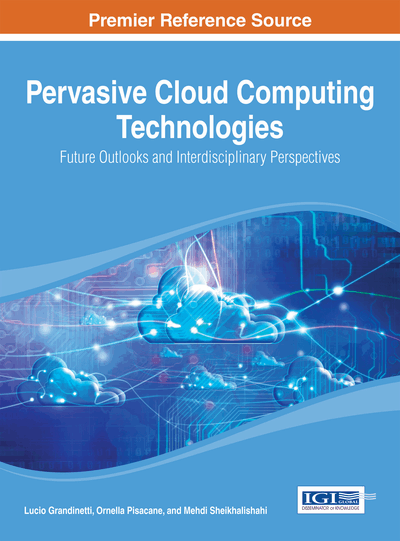Cloud Computing and Operations Research

DOI: 10.4018/978-1-4666-4683-4.ch010
Available
\$37.50
No Current Special Offers

Abstract

The aim of this chapter is twofold. On one hand, it shows how some classes of optimization problems can be efficiently solved on a cloud platform, especially in terms of storage capacity and computing power. Since an exhaustive treatment of this topic is beyond the purpose of the book, the attention is focused on the following classes of optimization problems: Linear Programming, Integer Linear Programming, Stochastic Optimization, and Logistics Management. On the other hand, the chapter also shows how some problems that arise in designing and managing the clouds can be mathematically formulated as optimization problems. Among these, the attention is focused on the Data Center Location Problem, the Virtual Machines Allocation Problem, and the Partner Provider Selection Problem. Finally, some useful conclusions are derived on the relation between Simulation-based Optimization and cloud computing.
Chapter Preview
Top

1. Introduction

Operations Research (OR) aims to define theoretical foundation and mathematical methods for modeling and simulation, of complex, challenging optimization, organization, management, planning problems and to devise the relevant computational algorithms and software for their numerical solution.

This chapter shows the close relation between cloud computing and OR. For this purpose, it is mainly organized in two parts.

The first part (Section 2) highlights how some classes of optimization problems can be efficiently solved by running the related algorithms on a cloud. However, since an exhaustive treatment of this topic lies outside the scope of this book, particular attention is given to the following classes of optimization problems: Linear Programming, Integer Linear Programming, Stochastic Optimization and Logistics Management. For a detailed introduction to Operations Research, we refer the interested readers to specific books. For each of the aforementioned classes, after introducing some basic concepts, major emphasis is then given to how they can be efficiently solved on a cloud by also providing application examples taken from literature. Finally, among the advantages, the possibility to use advanced optimization solvers and software tools as cloud services is also considered.

The second part of the chapter (Section 3), instead, describes how some issues that arise in designing and managing a cloud can be mathematically modeled as optimization problems. Particular attention will be given to some problems such as the Data Center Location Problem and the Virtual Machine Allocation Problem. Finally, the last two paragraphs of the chapter will be devoted to present some useful considerations on the problem of selecting the partner providers of a cloud and on the relation between Simulation-based Optimization approaches and cloud computing.

Top

2. Operations Research On Clouds

Generally speaking, the optimization problems aim to find the optimal solution (or a suboptimal one) among a set of feasible alternatives with reference to an objective function to be minimized or maximized. An example is to find the best resources utilization in order to reach a desired optimization objective (to minimize the total cost or maximize the service levels, for instance).

More precisely, the optimization problems are mathematically formulated by identifying the variables that represent the decisions to be determined and by defining the objective to be minimized or maximized, as well as the constraints on the decision variables, by functions on the variables domain.

Depending on the mathematical formulation, we can have different classes of optimization problems. After individuating the specific class, one can adopt the related solution approaches for the problem under investigation.

This section is mainly devoted to show how some of the most interesting classes of optimization problems can be efficiently solved by running the related solution algorithms on a cloud.

The optimization solvers are usually run on local computers that could not have the sufficient storage capability, for instance. To overcome these limitations, cloud computing can be seen as an effective solution. Among the other significant advantages, cloud computing can also provide the decision makers with the most advanced optimizers to be used as services.

The advantages provided by the adoption of a cloud platform can be more evident especially in the case of large scale optimization problems. It is worth noting that the term large scale is here referred not only to the optimization problems with a considerable number of variables and constraints but also to the ones that exhibit challenging structures for the currently available solution methods.

Complete Chapter List

Search this Book:
Reset